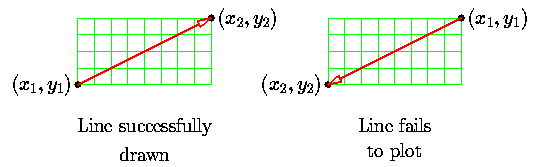Code for Program of Bresenham line drawing algorithm in C++ Programming

If we try out the C++ implementation of the Bresenham algorithm, we find it has some peculiar properties.
As expected, it fails to plot lines with negative slopes (try it and see what happens). It also fails to plot lines of positive slope greater than 1 (this is an interesting case, try it also and see if you can explain what is happening).
More unusually, we find that the order in which the endpoints are supplied to this routine is significant, it will only work as long as x1 is smaller than x2.
In fact, if we have two line segments with the same endpoints, and the same slope, this routine may draw one of them successfully but fails to draw the other one.Of course, this is not surprising really, when we consider that the function works by incrementing x. It does emphasise, however, that the routine is plotting vectors, direction is significant. Considering all the vectors from(x1,y1) to (x2,y2) we find that there are eight regions, (the ``octants'') and the basic Bresenham algorithm works in only one of them.
Output of This Program :